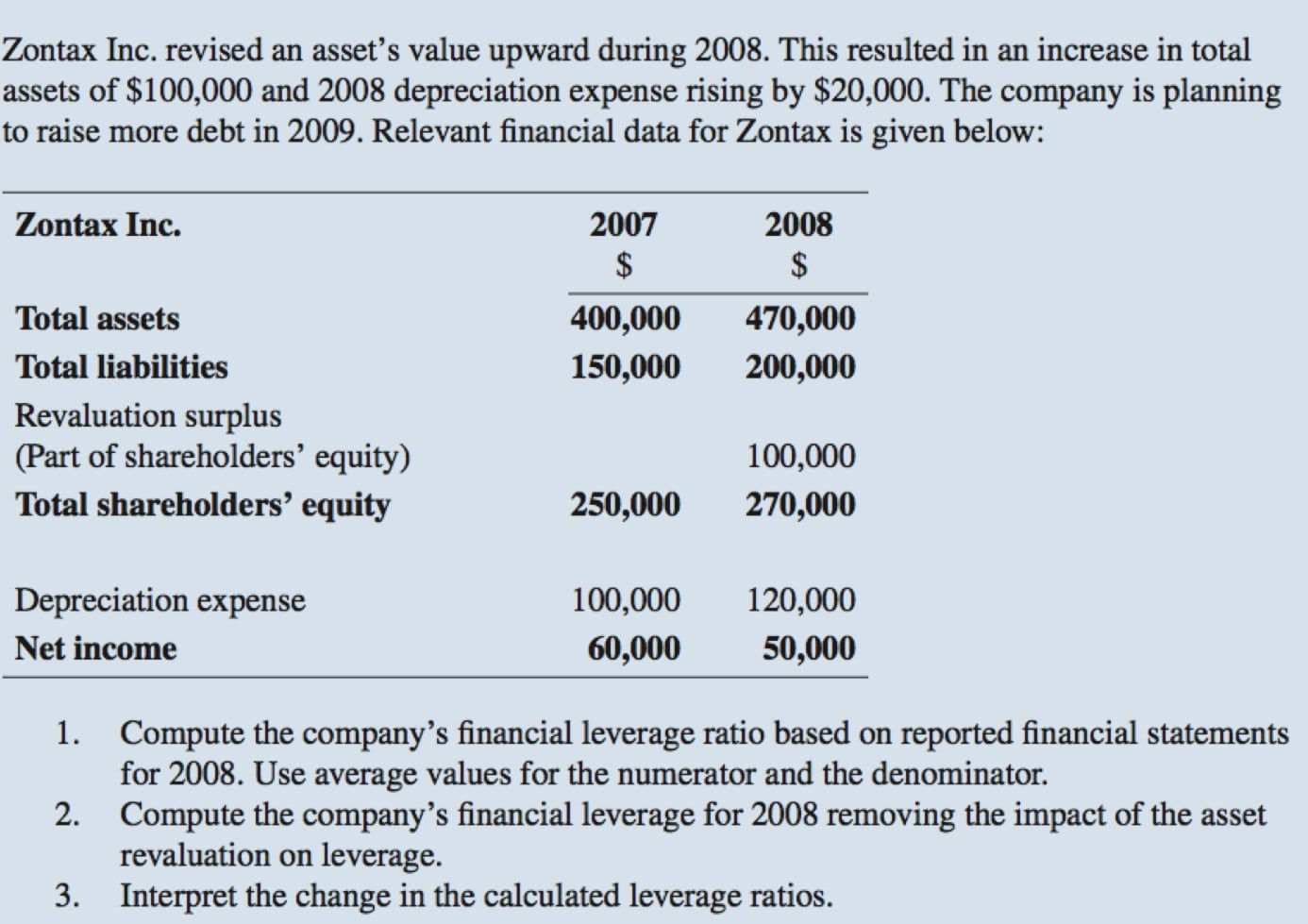CFA CFA Level 2 Revaluation model (IFRS)

# Revaluation model (IFRS)

• Author
Posts
• 18

Hi, can someone help to verify if my understanding on the  revaluation model is correct? When an asset is revalued higher than its historical cost, the gain is recorded on both the OCI (under revaluation surplus) and Balance Sheet (under shareholders’ equity in the revaluation surplus), correct?

• 4Also to follow up on the question on revaluation, the second question asks for financial leverage with asset revaluation removed from the calculation. In the answer sheet, the way to compute this is by removing the revaluation surplus and depreciation expense. I can understand how revaluation surplus and depreciation surplus change the value of “Average Total Asset” (numerator) but the solution also deduct the revaluation surplus and add the depreciation expense to the “Average Shareholders’ equity” (denominator). The formula to compute without the revaluation surplus is given as follow:

total asset with revaluation – valuation surplus + depreciation expense (divide by) total shareholders’ equity – valuation surplus + depreciation expense

my question is why do we include valuation surplus and depreciation surplus in computing the denominator?

thank you.

• 3

Hi there,

Here is the answer to your first question: “Can someone help to verify if my understanding on the  revaluation model is correct? When an asset is revalued higher than its historical cost, the gain is recorded on both the OCI (under revaluation surplus) and Balance Sheet (under shareholders’ equity in the revaluation surplus), correct?”

You are correct. However, in other problems there will be no account called Revaluation Surplus, it may go straight to Retained Earnings.

If the asset value (revaluation) > asset historical cost:

Assets increase by revaluation amount minus the extra accumulated depreciation
Equity increases by revaluation amount minus the extra accumulated depreciation
Net Income remains unchanged
OCI increases by the revaluation amount minus the extra accumulated depreciation

However, keep in mind that if asset value revaluation < asset historical cost (impairment) it will flow through the income statement.

• 2

Just for reference, here is the answer I got for question # 1

• 2

This is what I got for question #2. As far as why the increase in assets minus the extra depreciation is taken from the denominator is because a) the accounting equation needs to balance and b) because the amount of revaluation increases equity. I hope these answers help.